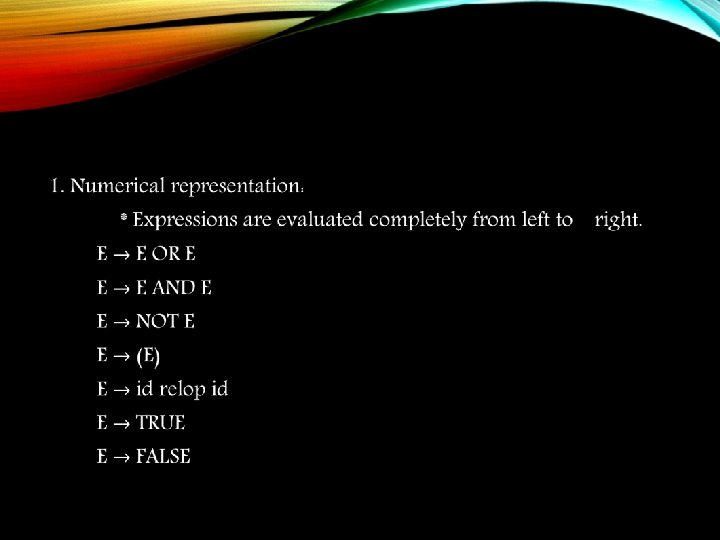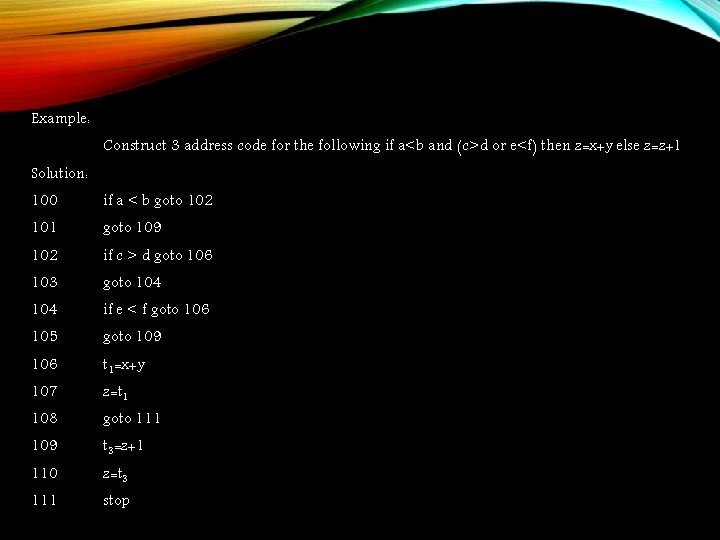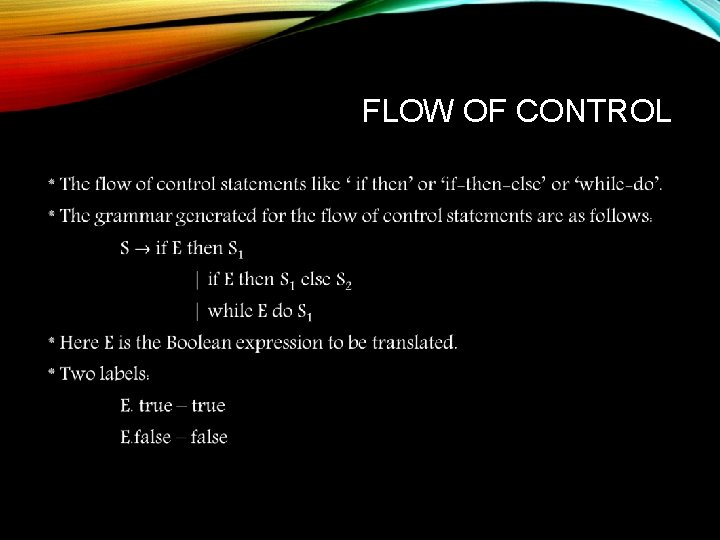# BOOLEAN EXPRESSIONS Boolean expressions are compared of the

• Slides: 7BOOLEAN EXPRESSIONS * Boolean expressions are compared of the Boolean operator applied between the variables or relational expressions. * Boolean expressions have two primary functions: 1. To compute logical expressions. 2. Used as condition expression. Ex: if then else, while Methods for translating Boolean expression: 1. Numerical representation 2. Flow of controlTRANSLATION SCHEME FOR BOOLEAN EXPRESSIONS Production Semantic action {E. place = newtemp(); append(E. place ‘=’ E 1. Place ‘or’ E 2. Place)} {E. place = newtemp(); append(E. place ‘=’ E 1. Place ‘and’ E 2. Place)} {E. place = newtemp(); append(E. place ‘=’ ‘not’ E 1. Place)} {E. place = newtemp(); E. place = E 1. place} {E. place = newtemp(); append(if id 1. place relop id 2. place ‘goto’ next_state + 3); append(E. place ‘=’ 0); append(goto next _state+2) append(E. place ‘=’ 1)} {E. place = newtemp(); append(E. place ‘=‘ 1)} {E. place = newtemp(); append(E. place ‘=‘ 0)}Example: Construct 3 address code for the following if a<b and (c>d or e<f) then z=x+y else z=z+1 Solution: 100 if a < b goto 102 101 goto 109 102 if c > d goto 106 103 goto 104 if e < f goto 106 105 goto 109 106 t 1=x+y 107 z=t 1 108 goto 111 109 t 3=z+1 110 z=t 3 111 stopFLOW OF CONTROL •Production Semantic rule E. true = newlabel() E. false = S. next S 1. Next = S. next S. code = E. code || gen(E. true ‘: ’)|| S 1. Code E. true = newlabel() E. false = newlabel() S 1. Next = S. next S 2. Next = S. next S. code = E. code || gen(E. true ‘: ’)|| S 1. code || gen(‘goto’ S. next)|| gen(E. false ‘: ’)|| S 2. code S. begin = newlabel(); E. true = newlabel(); E. false = S. next; S 1. Next = S. begin; S. code = gen(S. begin ‘: ’) || E. code || gen(E. true ‘: ’) || S 1. Code|| gen(‘goto’ S. begin)